# Precalculus : Approximate Area Using Rectangles

## Example Questions

### Example Question #1 : Area Under A Curve

Approximate the area under the following curve fromtousing a midpoint Riemann sum with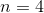: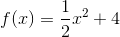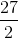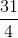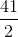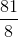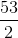Explanation:

If we want to approximate the area under a curve using n=4, that means we will be using 4 rectangles. Because the problem asks us to approximate the area from x=0 to x=4, this means we will have a rectangle between x=0 and x=1, between x=1 and x=2, between x=2 and x=3, and between x=3 and x=4. We want to use a midpoint Riemann sum, so the height of each rectangle will be the value of the function at the midpoint of each interval: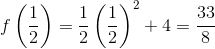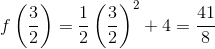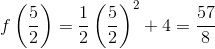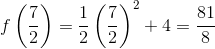Now that we know the height of each rectangle, all we have to do is find its area by multiplying the height by the width, which is just 1 for each rectangle. Then we add the area of all the rectangles to find our approximation for the area under the curve from x=0 to x=4: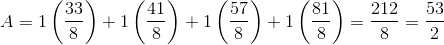### Example Question #1 : Area Under A Curve

Using a left hand approximation andrectangles, what is the area under the curve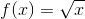on the interval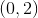?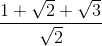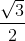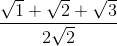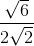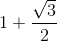Explanation:

A left hand approximation requires us to slice the region into four rectangles of equal width. Since the interval on which we are slicing isand we want to create four rectangles of equal width, the width of each rectangle must be,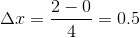.

The height of each rectangular slice is given by the function value at the left edge of each rectangle. Beginning at the leftmost edge of the first rectangle on the interval, these left endpoints exist at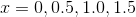, but not atas that is the right edge of the fourth and final rectangle on the interval.

Using the function given,, the first rectangle has height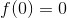. The rest have height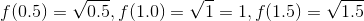.

Multiply each by the width of each rectangle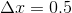to get the area of each rectangular slice. Then add the area of the slices together, and simplify to get the correct result.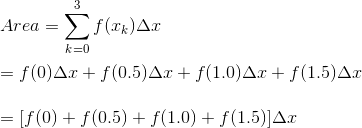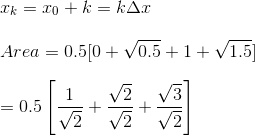### Example Question #178 : Introductory Calculus

Let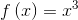Approximate the area underneath the function on the interval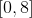divided into four sub-intervals using the midpoint height of a rectangle for each sub-interval.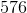units squared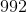units squared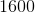units squared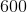units squaredunits squared

Explanation:

The intervaldivided into four sub-intervals gives rectangles with vertices of the bases at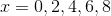For the approximated area, we need to find the rectangle heights which values come from the midpoint of the sub-intervals, or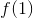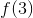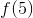, and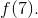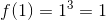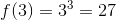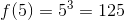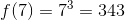Because each sub-interval has width, the approximated area using rectangles is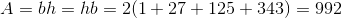As such, the approximated area isunits squared

### Example Question #1 : Area Under A Curve

Let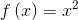Approximate the area underneath the function on the interval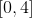divided into two sub-intervals using the midpoint height of a rectangle for each sub-interval.units squaredunits squaredunits squaredunits squaredunits squared

Explanation:

The intervaldivided into two sub-intervals gives two rectangles with vertices of the bases at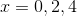For the approximated area, we need to find the rectangle heights which values come from the midpoint of the sub-intervals, orand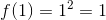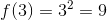Because each sub-interval has width, the approximated area using rectangles is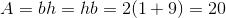As such, the approximated area isunits squared

### Example Question #1 : Approximate Area Using Rectangles

Approximate the area under the curve given by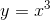on the interval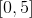using left endpoints.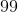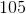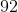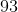Explanation:

In order to approximate the area under a curve using rectangles, one must take the sum of the areas of discrete rectangles under the curve. Taking the height of each rectangle as the function evaluated at the left endpoint, we obtain the following rectangle areas: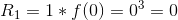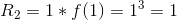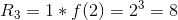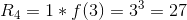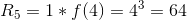The sum of the individual rectangles yields an overall area approximation of 100.

### Example Question #1 : Approximate Area Using Rectangles

Approximate the area under the curve given byon the intervalusing right endpoints.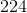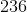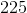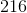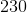Explanation:

In order to approximate the area under a curve using rectangles, one must take the sum of the areas of discrete rectangles under the curve. Taking the height of each rectangle as the function evaluated at the right endpoint, we obtain the following rectangle areas: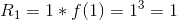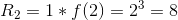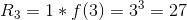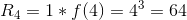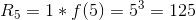The sum of the individual rectangles yields an overall area approximation of 225.

### All Precalculus Resources